Documentation

# fevd

Generate vector autoregression (VAR) model forecast error variance decomposition (FEVD)

## Syntax

``Decomposition = fevd(Mdl)``
``Decomposition = fevd(Mdl,Name,Value)``
``[Decomposition,Lower,Upper] = fevd(___)``

## Description

The `fevd` function returns the forecast error decomposition (FEVD) of the variables in a VAR(p) model attributable to shocks to each response variable in the system. A fully specified `varm` model object characterizes the VAR model.

To estimate or plot the FEVD of a dynamic linear model characterized by structural, autoregression, or moving average coefficient matrices, see `armafevd`.

The FEVD provides information about the relative importance of each innovation in affecting the forecast error variance of all response variables in the system. In contrast, the impulse response function (IRF) traces the effects of an innovation shock to one variable on the response of all variables in the system. To estimate the IRF of a VAR model characterized by a `varm` model object, see `irf`.

example

````Decomposition = fevd(Mdl)` returns the orthogonalized FEVDs of the response variables that compose the VAR(p) model `Mdl`, characterized by a fully specified `varm` model object. `fevd` shocks variables at time 0, and returns the FEVD for times 1 through 20.```

example

````Decomposition = fevd(Mdl,Name,Value)` uses additional options specified by one or more name-value pair arguments. For example, `'NumObs',10,'Method',"generalized"` specifies estimating a generalized FEVD for periods 1 through 10.```

example

````[Decomposition,Lower,Upper] = fevd(___)` uses any of the input argument combinations in the previous syntaxes and returns lower and upper 95% confidence bounds for each period and variable in the FEVD. If you specify series of residuals by using the `E` name-value pair argument, then `fevd` estimates the confidence bounds by bootstrapping the specified residuals.Otherwise, `fevd` estimates confidence bounds by conducting Monte Carlo simulation. If `Mdl` is a custom `varm` model object (an object not returned by `estimate` or modified after estimation), `fevd` might require a sample size for the simulation `SampleSize` or presample responses `Y0`.```

## Examples

collapse all

Fit a 4-D VAR(2) model to Danish money and income rate series. Then, estimate and plot the orthogonalized FEVD from the estimated model.

Load the Danish money and income data set.

`load Data_JDanish`

The data set includes four times series in the table `DataTable`. For more details on the data set, enter `Description` at the command line.

Assuming that the series are stationary, create a `varm` model object that represents a 4-D VAR(2) model. Specify the variable names.

```Mdl = varm(4,2); Mdl.SeriesNames = DataTable.Properties.VariableNames;```

`Mdl` is a `varm` model object specifying the structure of a 4-D VAR(2) model; it is a template for estimation.

Fit the VAR(2) model to the data set.

`Mdl = estimate(Mdl,DataTable.Series);`

`Mdl` is a fully specified `varm` model object representing an estimated 4-D VAR(2) model.

Estimate the orthogonalized FEVD from the estimated VAR(2) model.

`Decomposition = fevd(Mdl);`

`Decomposition` is a 20-by-4-by-4 array representing the FEVD of `Mdl`. Rows correspond to consecutive time points from time 1 to 20, columns correspond to variables receiving a one-standard-deviation innovation shock at time 0, and pages correspond to the variables whose forecast error variance `fevd` decomposes. `Mdl.SeriesNames` specifies the variable order.

Because `Decomposition` represents an orthogonalized FEVD, rows should sum to `1`. This characteristic illustrates that orthogonalized FEVDs represent proportions of variance contributions. Confirm that all rows of `Decomposition` sum to `1`.

```rowsums = sum(Decomposition,2); sum((rowsums - 1).^2 > eps)```
```ans = ans(:,:,1) = 0 ans(:,:,2) = 0 ans(:,:,3) = 0 ans(:,:,4) = 0 ```

Row sums among the pages are close to 1.

Display the contributions to the forecast error variance of the bond rate when real income is shocked at time 0.

`Decomposition(:,2,3)`
```ans = 20×1 0.0499 0.1389 0.1700 0.1807 0.1777 0.1694 0.1601 0.1516 0.1446 0.1390 ⋮ ```

Plot the FEVDs of all series on separate plots by passing the estimated AR coefficient matrices and innovations covariance matrix of `Mdl` to `armafevd`.

`armafevd(Mdl.AR,[],"InnovCov",Mdl.Covariance);`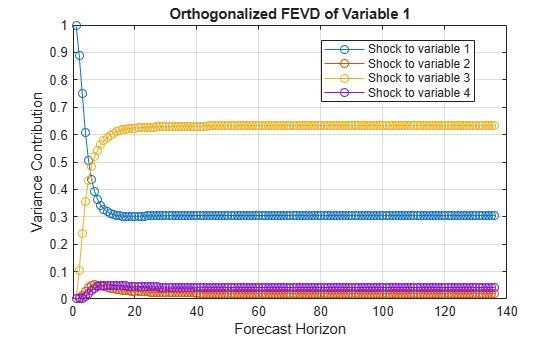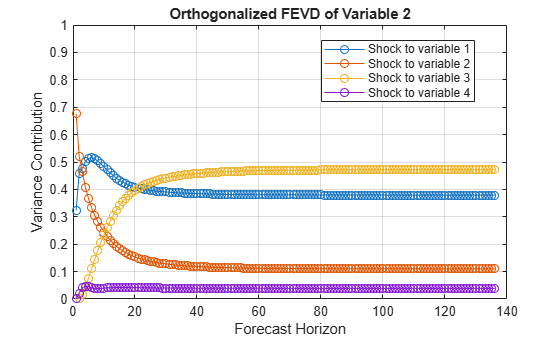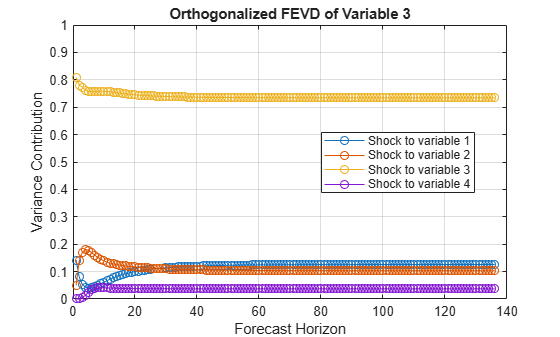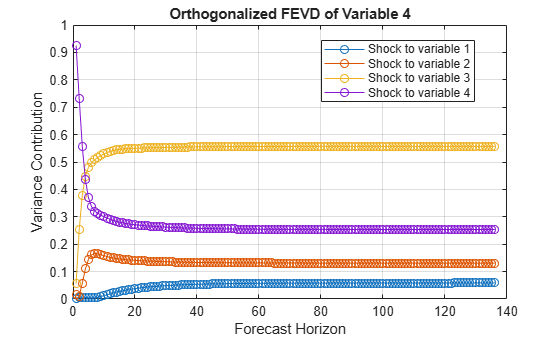Each plot shows the four FEVDs of a variable when all other variables are shocked at time 0. `Mdl.SeriesNames` specifies the variable order.

Consider the 4-D VAR(2) model in Estimate and Plot VAR Model FEVD. Estimate the generalized FEVD of the system for 100 periods.

Load the Danish money and income data set, then estimate the VAR(2) model.

```load Data_JDanish Mdl = varm(4,2); Mdl.SeriesNames = DataTable.Properties.VariableNames; Mdl = estimate(Mdl,DataTable.Series);```

Estimate the generalized FEVD from the estimated VAR(2) model over a forecast horizon with length 100.

`Decomposition = fevd(Mdl,"Method","generalized","NumObs",100);`

`Decomposition` is a 100-by-4-by-4 array representing the generalized FEVD of `Mdl`.

Plot the generalized FEVD of the bond rate when real income is shocked at time 0.

```figure; plot(1:100,Decomposition(:,2,3)) title("FEVD of IB When Y Is Shocked") xlabel("Forecast Horizon") ylabel("Variance Contribution") grid on```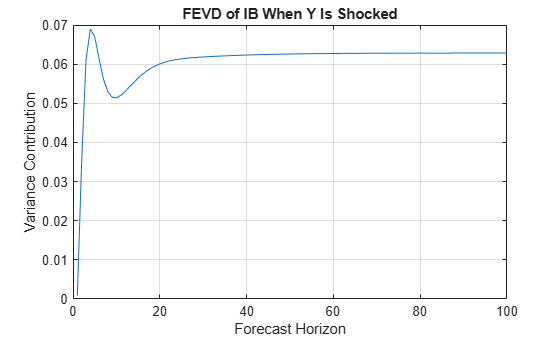When real income is shocked, the contribution of the bond rate to the forecast error variance settles at approximately 0.061.

Consider the 4-D VAR(2) model in Estimate and Plot VAR Model FEVD. Estimate and plot its orthogonalized FEVD and 95% Monte Carlo confidence intervals on the true FEVD.

Load the Danish money and income data set, then estimate the VAR(2) model.

```load Data_JDanish Mdl = varm(4,2); Mdl.SeriesNames = DataTable.Properties.VariableNames; Mdl = estimate(Mdl,DataTable.Series);```

Estimate the FEVD and corresponding 95% Monte Carlo confidence intervals from the estimated VAR(2) model.

```rng(1); % For reproducibility [Decomposition,Lower,Upper] = fevd(Mdl);```

`Decomposition`, `Lower`, and `Upper` are 20-by-4-by-4 arrays representing the orthogonalized FEVD of `Mdl` and corresponding lower and upper bounds of the confidence intervals. For all arrays, rows correspond to consecutive time points from time 1 to 20, columns correspond to variables receiving a one-standard-deviation innovation shock at time 0, and pages correspond to the variables whose forecast error variance `fevd` decomposes. `Mdl.SeriesNames` specifies the variable order.

Plot the orthogonalized FEVD with its confidence bounds of the bond rate when real income is shocked at time 0.

```fevdshock2resp3 = Decomposition(:,2,3); FEVDCIShock2Resp3 = [Lower(:,2,3) Upper(:,2,3)]; figure; h1 = plot(1:20,fevdshock2resp3); hold on h2 = plot(1:20,FEVDCIShock2Resp3,'r--'); legend([h1 h2(1)],["FEVD" "95% Confidence Interval"],... 'Location',"best") xlabel("Forecast Horizon"); ylabel("Variance Contribution"); title("FEVD of IB When Y Is Shocked"); grid on hold off```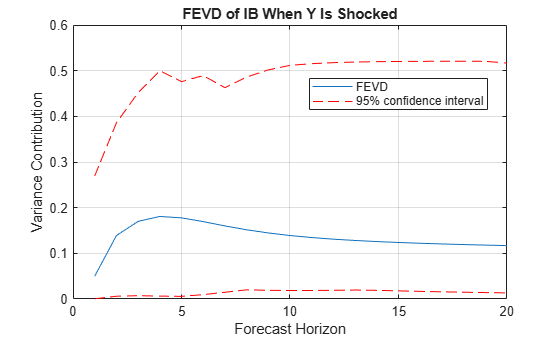In the long run, and when real income is shocked, the proportion of forecast error variance of the bond rate settles between approximately 0 and 0.5 with 95% confidence.

Consider the 4-D VAR(2) model in Estimate and Plot VAR Model FEVD. Estimate and plot its orthogonalized FEVD and 90% bootstrap confidence intervals on the true FEVD.

Load the Danish money and income data set, then estimate the VAR(2) model. Return the residuals from model estimation.

```load Data_JDanish Mdl = varm(4,2); Mdl.SeriesNames = DataTable.Properties.VariableNames; [Mdl,~,~,E] = estimate(Mdl,DataTable.Series); T = size(DataTable,1) % Total sample size```
```T = 55 ```
`n = size(E,1) % Effective sample size`
```n = 53 ```

`E` is a 53-by-4 array of residuals. Columns correspond to the variables in `Mdl.SeriesNames`. The `estimate` function requires `Mdl.P` = 2 observations to initialize a VAR(2) model for estimation. Because presample data (`Y0`) is unspecified, `estimate` takes the first two observations in the specified response data to initialize the model. Therefore, the resulting effective sample size is `T``Mdl.P` = 53, and rows of `E` correspond to the observation indices 3 through `T`.

Estimate the orthogonalized FEVD and corresponding 90% bootstrap confidence intervals from the estimated VAR(2) model. Draw 500 paths of length `n` from the series of residuals.

```rng(1); % For reproducibility [Decomposition,Lower,Upper] = fevd(Mdl,"E",E,"NumPaths",500,... "Confidence",0.9);```

Plot the orthogonalized FEVD with its confidence bounds of the bond rate when real income is shocked at time 0.

```fevdshock2resp3 = Decomposition(:,2,3); FEVDCIShock2Resp3 = [Lower(:,2,3) Upper(:,2,3)]; figure; h1 = plot(0:19,fevdshock2resp3); hold on h2 = plot(0:19,FEVDCIShock2Resp3,'r--'); legend([h1 h2(1)],["FEVD" "90% Confidence Interval"],... 'Location',"best") xlabel("Time Index"); ylabel("Response"); title("FEVD of IB When Y Is Shocked"); grid on hold off```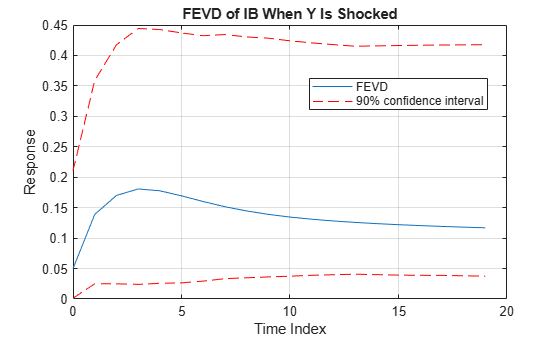In the long run, and when real income is shocked, the proportion of forecast error variance of the bond rate settles between approximately 0.05 and 0.4 with 90% confidence.

## Input Arguments

collapse all

VAR model, specified as a `varm` model object created by `varm` or `estimate`. `Mdl` must be fully specified.

### Name-Value Pair Arguments

Specify optional comma-separated pairs of `Name,Value` arguments. `Name` is the argument name and `Value` is the corresponding value. `Name` must appear inside quotes. You can specify several name and value pair arguments in any order as `Name1,Value1,...,NameN,ValueN`.

Example: `'NumObs',10,'Method',"generalized"` specifies estimating a generalized FEVD for periods 1 through 10.

#### Options for All FEVDs

collapse all

Number of periods for which `fevd` computes the FEVD (the forecast horizon), specified as the comma-separated pair consisting of `'NumObs'` and a positive integer. `NumObs` specifies the number of observations to include in the FEVD (the number of rows in `Decomposition`).

Example: `'NumObs',10` specifies estimation of the FEVD for times 1 through 10.

Data Types: `double`

FEVD computation method, specified as the comma-separated pair consisting of `'Method'` and a value in this table.

ValueDescription
`"orthogonalized"`Compute variance decompositions using orthogonalized, one-standard-deviation innovation shocks. `fevd` uses the Cholesky factorization of `Mdl.Covariance` for orthogonalization.
`"generalized"`Compute variance decompositions using one-standard-deviation innovation shocks.

Example: `'Method',"generalized"`

Data Types: `char` | `string`

#### Options for Confidence Bound Estimation

collapse all

Number of sample paths (trials) to generate, specified as the comma-separated pair consisting of `'NumPaths'` and a positive integer.

Example: `'NumPaths',1000` generates `1000` sample paths from which the software derives the confidence bounds.

Data Types: `double`

Number of observations for the Monte Carlo simulation or bootstrap per sample path, specified as the comma-separated pair consisting of `'SampleSize'` and a positive integer.

• If `Mdl` is an estimated `varm` model object (an object returned by `estimate` and unmodified thereafter), then the default is the sample size of the data to which the model is fit (see `summarize`).

• If `fevd` estimates confidence bounds by conducting a Monte Carlo simulation (for details, see `E`), you must specify `SampleSize`.

• If `fevd` estimates confidence bounds by bootstrapping residuals, the default is the length of the specified series of residuals (`size(E,1)`).

Example: If you specify `'SampleSize',100` and do not specify the `'E'` name-value pair argument, the software estimates confidence bounds from `NumPaths` random paths of length `100` from `Mdl`.

Example: If you specify `'SampleSize',100,'E',E`, the software resamples, with replacement, `100` observations (rows) from `E` to form a sample path of innovations to filter through `Mdl`. The software forms `NumPaths` random sample paths from which it derives confidence bounds.

Data Types: `double`

Presample response data that provides initial values for model estimation during the simulation, specified as the comma-separated pair consisting of `'Y0'` and a `numpreobs`-by-`numseries` numeric matrix.

Rows of `Y0` correspond to periods in the presample, and the last row contains the latest presample response. `numpreobs` is the number of specified presample responses and it must be at least `Mdl.P`. If `numpreobs` exceeds `Mdl.P`, then `fevd` uses only the latest `Mdl.P` rows.

`numseries` is the dimensionality of the input VAR model `Mdl.NumSeries`. Columns must correspond to the response variables in `Mdl.SeriesNames`.

• If `Mdl` is an estimated `varm` model object (an object returned by `estimate` and unmodified thereafter), `fevd` sets `Y0` to the presample response data used for estimation by default (see `'Y0'`).

• Otherwise, you must specify `Y0`.

Data Types: `double`

Predictor data for estimating the model regression component during the simulation, specified as the comma-separated pair consisting of `'X'` and a numeric matrix containing `numpreds` columns.

`numpreds` is the number of predictor variables (`size(Mdl.Beta,2)`).

Rows correspond to observations. `X` must have at least `SampleSize` rows. If you supply more rows than necessary, `fevd` uses only the latest `SampleSize` observations. The last row contains the latest observation.

Columns correspond to individual predictor variables. All predictor variables are present in the regression component of each response equation.

To maintain model consistency when `fevd` estimates the confidence bounds, a good practice is to specify `X` when `Mdl` has a regression component. If `Mdl` is an estimated model, specify the predictor data used during model estimation (see `'X'`).

By default, `fevd` excludes the regression component from confidence bound estimation, regardless of its presence in `Mdl`.

Data Types: `double`

Series of residuals from which to draw bootstrap samples, specified as the comma-separated pair consisting of `'E'` and a numeric matrix containing `numseries` columns. `fevd` assumes that `E` is free of serial correlation.

Columns contain the residual series corresponding to the response series names in `Mdl.SeriesNames`.

If `Mdl` is an estimated `varm` model object (an object returned by `estimate`), you can specify `E` as the inferred residuals from estimation (see `E` or `infer`).

By default, `fevd` derives confidence bounds by conducting a Monte Carlo simulation.

Data Types: `double`

Confidence level for confidence bounds, specified as the comma-separated pair consisting of `'Confidence'` and a numeric scalar in [0,1].

Suppose `Confidence` = c. Then, 100(1 – c)/2 percent of the impulse responses lie outside the confidence bounds.

The default value is `0.95`, which implies that the confidence bounds represent 95% confidence intervals.

Data Types: `double`

## Output Arguments

collapse all

FEVD of each response variable, returned as a `numobs`-by-`numseries`-by-`numseries` numeric array. `numobs` is the value of `NumObs`. Columns and pages correspond to the response variables in `Mdl.SeriesNames`.

`Decomposition(t,j,k)` is the contribution to the variance decomposition of variable `k` attributable to a one-standard-deviation innovation shock to variable `j` at time `t`, for `t` = 1,2,…,`numobs`, `j` = 1,2,...,`numseries`, and `k` = 1,2,...,`numseries`.

Lower confidence bounds, returned as a `numobs`-by-`numseries`-by-`numseries` numeric array. Elements of `Lower` correspond to elements of `Decomposition`.

`Lower(t,j,k)` is the lower bound of the `100*Confidence`% percentile interval on the true contribution to the variance decomposition of variable `k` attributable to a one-standard-deviation innovation shock to variable `j` at time 0.

Upper confidence bounds, returned as a `numobs`-by-`numseries`-by-`numseries` numeric array. Elements of `Upper` correspond to elements of `Decomposition`.

`Upper(t,j,k)` is the upper bound of the `100*Confidence`% percentile interval on the true contribution to the variance decomposition of variable `k` attributable to a one-standard-deviation innovation shock to variable `j` at time 0.

collapse all

### Forecast Error Variance Decomposition

The forecast error variance decomposition (FEVD) of a multivariate, dynamic system shows the relative importance of a shock to each innovation in affecting the forecast error variance of all variables in the system.

Consider a `numseries`-D VAR(p) model for the multivariate response variable yt. In lag operator notation, the infinite lag MA representation of yt is:

`$\begin{array}{c}{y}_{t}={\Phi }^{-1}\left(L\right)\left(c+\beta {x}_{t}+\delta t\right)+{\Phi }^{-1}\left(L\right){\epsilon }_{t}\\ =\Omega \left(L\right)\left(c+\beta {x}_{t}+\delta t\right)+\Omega \left(L\right){\epsilon }_{t}.\end{array}$`

The general form of the FEVD of ykt (variable k) m periods into the future, attributable to a one-standard-deviation innovation shock to yjt, is

`${\gamma }_{mjk}=\frac{\sum _{t=0}^{m-1}{\left({e}_{k}{}^{\prime }{C}_{t}{e}_{j}\right)}^{2}}{\sum _{t=0}^{m-1}{e}_{k}^{\prime }{\Omega }_{t}\Sigma {\Omega }_{t}^{\prime }{e}_{k}}.$`
• ej is a selection vector of length `numseries` containing a 1 in element j and zeros elsewhere.

• For orthogonalized FEVDs, ${C}_{m}={\Omega }_{m}P,$ where P is the lower triangular factor in the Cholesky factorization of Σ.

• For generalized FEVDs, ${C}_{m}={\sigma }_{j}^{-1}{\Omega }_{m}\Sigma ,$ where σj is the standard deviation of innovation j.

• The numerator is the contribution of an innovation shock to variable j to the forecast error variance of the m-step-ahead forecast of variable k. The denominator is the mean square error (MSE) of the m-step-ahead forecast of variable k .

### Vector Autoregression Model

A vector autoregression (VAR) model is a stationary multivariate time series model consisting of a system of m equations of m distinct response variables as linear functions of lagged responses and other terms.

A VAR(p) model in difference-equation notation and in reduced form is

`${y}_{t}=c+{\Phi }_{1}{y}_{t-1}+{\Phi }_{2}{y}_{t-2}+...+{\Phi }_{p}{y}_{t-p}+\beta {x}_{t}+\delta t+{\epsilon }_{t}.$`

• yt is a `numseries`-by-1 vector of values corresponding to `numseries` response variables at time t, where t = 1,...,T. The structural coefficient is the identity matrix.

• c is a `numseries`-by-1 vector of constants.

• Φj is a `numseries`-by-`numseries` matrix of autoregressive coefficients, where j = 1,...,p and Φp is not a matrix containing only zeros.

• xt is a `numpreds`-by-1 vector of values corresponding to `numpreds` exogenous predictor variables.

• β is a `numseries`-by-`numpreds` matrix of regression coefficients.

• δ is a `numseries`-by-1 vector of linear time-trend values.

• εt is a `numseries`-by-1 vector of random Gaussian innovations, each with a mean of 0 and collectively a `numseries`-by-`numseries` covariance matrix Σ. For ts, εt and εs are independent.

Condensed and in lag operator notation, the system is

`$\Phi \left(L\right){y}_{t}=c+\beta {x}_{t}+\delta t+{\epsilon }_{t},$`

where $\Phi \left(L\right)=I-{\Phi }_{1}L-{\Phi }_{2}{L}^{2}-...-{\Phi }_{p}{L}^{p}$, Φ(L)yt is the multivariate autoregressive polynomial, and I is the `numseries`-by-`numseries` identity matrix.

## Algorithms

• If `Method` is `"orthogonalized"`, then `fevd` orthogonalizes the innovation shocks by applying the Cholesky factorization of the model covariance matrix `Mdl.Covariance`. The covariance of the orthogonalized innovation shocks is the identity matrix, and the FEVD of each variable sums to one (that is, the sum along any row of `Decomposition` is one). Therefore, the orthogonalized FEVD represents the proportion of forecast error variance attributable to various shocks in the system. However, the orthogonalized FEVD generally depends on the order of the variables.

If `Method` is `"generalized"`, then the resulting FEVD is invariant to the order of the variables, and is not based on an orthogonal transformation. Also, the resulting FEVD sums to one for a particular variable only when `Mdl.Covariance` is diagonal . Therefore, the generalized FEVD represents the contribution to the forecast error variance of equation-wise shocks to the response variables in the model.

• If `Mdl.Covariance` is a diagonal matrix, then the resulting generalized and orthogonalized FEVDs are identical. Otherwise, the resulting generalized and orthogonalized FEVDs are identical only when the first variable shocks all variables (that is, all else being the same, both methods yield the same value of `Decomposition(:,1,:)`).

• `NaN` values in `Y0`, `X`, and `E` indicate missing data. `fevd` removes missing data from these arguments by list-wise deletion. Each argument, if a row contains at least one `NaN`, then `fevd` removes the entire row.

List-wise deletion reduces the sample size, can create irregular time series, and can cause `E` and `X` to be unsynchronized.

• The predictor data `X` represents a single path of exogenous multivariate time series. If you specify `X` and the VAR model `Mdl` has a regression component (`Mdl.Beta` is not an empty array), `fevd` applies the same exogenous data to all paths used for confidence interval estimation.

• `fevd` conducts a simulation to estimate the confidence bounds `Lower` and `Upper`.

• If you do not specify residuals `E`, then `fevd` conducts a Monte Carlo simulation by following this procedure:

1. Simulate `NumPaths` response paths of length `SampleSize` from `Mdl`.

2. Fit `NumPaths` models that have the same structure as `Mdl` to the simulated response paths. If `Mdl` contains a regression component and you specify `X`, the `fevd` fits the `NumPaths` models to the simulated response paths and `X` (the same predictor data for all paths).

3. Estimate `NumPaths` FEVDs from the `NumPaths` estimated models.

4. For each time point t = 0,…,`NumObs`, estimate the confidence intervals by computing 1 – `Confidence` and `Confidence` quantiles (the upper and lower bounds, respectively).

• If you specify residuals `E`, then `fevd` conducts a nonparametric bootstrap by following this procedure:

1. Resample, with replacement, `SampleSize` residuals from `E`. Perform this step `NumPaths` times to obtain `NumPaths` paths.

2. Center each path of bootstrapped residuals.

3. Filter each path of centered, bootstrapped residuals through `Mdl` to obtain `NumPaths` bootstrapped response paths of length `SampleSize`.

4. Complete steps 2 through 4 of the Monte Carlo simulation, but replace the simulated response paths with the bootstrapped response paths.

 Hamilton, J. D. Time Series Analysis. Princeton, NJ: Princeton University Press, 1994.

 Lütkepohl, H. "Asymptotic Distributions of Impulse Response Functions and Forecast Error Variance Decompositions of Vector Autoregressive Models." Review of Economics and Statistics. Vol. 72, 1990, pp. 116–125.

 Lütkepohl, H. New Introduction to Multiple Time Series Analysis. New York, NY: Springer-Verlag, 2007.

 Pesaran, H. H., and Y. Shin. "Generalized Impulse Response Analysis in Linear Multivariate Models." Economic Letters. Vol. 58, 1998, pp. 17–29.